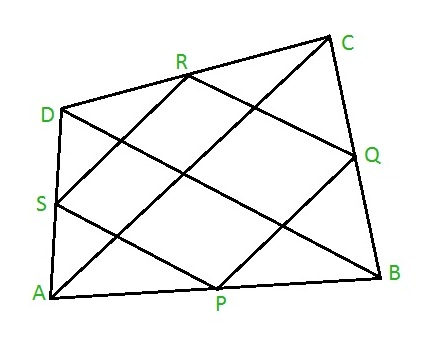# Perimeter and Area of Varignon’s Parallelogram

Given a and b are the lengths of the diagonals AC and BD of a quadrilateral ABCD with the area of quadrilateral as s. The task is to find the perimeter and area of the Varignon’s parallelogram PQRS.

Note: When we join the mid-points of the sides of any quadrilateral, the new quadrilateral formed inside will always be a parallelogram and this paralleogram is known as the Varignon’s paralleogram named upon the French Mathematician Pierre Varignon. Thus, PQRS will be a parallelogram since it is formed by joining the mid-points of quadrilateral ABCD as shown below:Example:

Input: a = 7, b = 8, s = 10
Output: Perimeter = 15, Area = 5

## Recommended: Please try your approach on {IDE} first, before moving on to the solution.

Approach: The perimeter of Varignon’s parallelogram PQRS is equal to the sum of the length of the diagonals of quadrilateral ABCD.
Hence, Perimeter = a + b, where a and b are lengths of diagonals AC and BD.
Also, the area of the Varignon’s parallelogram is always half the area of quadrilateral ABCD.
Hence, Area = s / 2, where s is the area of quadrilateral ABCD.

Below is the implementation of the above approach:

## C

 `// C program to find the perimeter and area ` `#include ` ` `  `// Function to find the perimeter ` `float` `per( ``float` `a, ``float` `b ) ` `{ ` `    ``return` `( a + b ); ` `} ` ` `  `// Function to find the area ` `float` `area( ``float` `s ) ` `{ ` `    ``return` `( s/2 ); ` `} ` ` `  `// Driver code ` `int` `main() ` `{ ` `    ``float` `a = 7, b = 8, s = 10; ` `    ``printf``(``"%f\n"``, ` `           ``per( a, b )); ` `    ``printf``(``"%f"``, ` `           ``area( s )); ` `    ``return` `0; ` `} `

## Java

 `// Java code to find the perimeter and area ` `import` `java.lang.*; ` ` `  `class` `GFG { ` ` `  `    ``// Function to find the perimeter ` `    ``public` `static` `double` `per(``double` `a, ``double` `b) ` `    ``{ ` `        ``return` `(a + b); ` `    ``} ` `    ``// Function to find the area ` `    ``public` `static` `double` `area(``double` `s) ` `    ``{ ` `        ``return` `(s / ``2``); ` `    ``} ` ` `  `    ``// Driver code ` `    ``public` `static` `void` `main(String[] args) ` `    ``{ ` `        ``double` `a = ``7``, b = ``8``, s = ``10``; ` `        ``System.out.println(per(a, b)); ` `        ``System.out.println(area(s)); ` `    ``} ` `} `

## Python3

 `# Python3 code to find the perimeter and area ` `     `  `# Function to find the perimeter ` `def` `per( a, b ): ` `    ``return` `( a ``+` `b ) ` `# Function to find the area ` `def` `area( s ): ` `    ``return` `( s ``/` `2` `) ` `     `  `# Driver code ` `a ``=` `7` `b ``=` `8` `s ``=` `10` `print``( per( a, b )) ` `print``( area( s )) `

## C#

 `// C# code to find the perimeter and area ` `using` `System; ` ` `  `class` `GFG { ` ` `  `    ``// Function to find the perimeter ` `    ``public` `static` `double` `per(``double` `a, ``double` `b) ` `    ``{ ` `        ``return` `(a + b); ` `    ``} ` `    ``// Function to find the area ` `    ``public` `static` `double` `area(``double` `s) ` `    ``{ ` `        ``return` `(s / 2); ` `    ``} ` ` `  `    ``// Driver code ` `    ``public` `static` `void` `Main() ` `    ``{ ` `        ``double` `a = 7.0, b = 8.0, s = 10.0; ` `        ``Console.WriteLine(per(a, b)); ` `        ``Console.Write(area(s)); ` `    ``} ` `} `

## PHP

 ` `

Output:

```15
5
```

Attention reader! Don’t stop learning now. Get hold of all the important DSA concepts with the DSA Self Paced Course at a student-friendly price and become industry ready.

My Personal Notes arrow_drop_upCheck out this Author's contributed articles.

If you like GeeksforGeeks and would like to contribute, you can also write an article using contribute.geeksforgeeks.org or mail your article to contribute@geeksforgeeks.org. See your article appearing on the GeeksforGeeks main page and help other Geeks.

Please Improve this article if you find anything incorrect by clicking on the "Improve Article" button below.